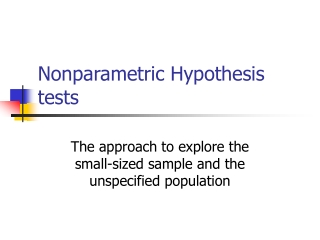DownloadDownload PresentationNonparametric Hypothesis tests

Nonparametric Hypothesis tests

Télécharger la présentationNonparametric Hypothesis tests

- - - - - - - - - - - - - - - - - - - - - - - - - - - E N D - - - - - - - - - - - - - - - - - - - - - - - - - - -
Presentation Transcript

1. Nonparametric Hypothesis tests The approach to explore the small-sized sample and the unspecified population

2. Nonparametric problems • No proper distribution for hypothesis test • Too small size to adopt the normality approximation • Most nonparametric methods based on ranks instead of original data

3. Some nonparametric tests • The sign test • Testing the median value • The signed rank test • Testing the symmetry of distribution • The rank sum test • Testing the similarity between two populations • The runs test • Testing the randomness of distribution

4. The sign test (i)

5. The signed rank test (i) in the ascending order

6. The signed rank test (ii)

7. The signed rank test (iii)

8. The signed rank test (iv)

9. The signed rank test (v)

10. The rank sum test (i)

11. The rank sum test (ii)

12. The rank sum test (iii)—another statistics

13. The rank sum test (iv)—approximation approach

14. The runs test (i)

15. The runs test (ii)

16. The runs test (iii) =2*the smaller number+1

17. The runs test (iv)

18. Example P-value for randomness of this sequence? =2*min(PH0{R≧3},PH0{R≦3}) 2≦R≦7

19. The runs test (v)

20. Some famous nonparametric statistical methods • Wilcoxon signed rank test for the symmetric distribution • Wilcoxon, Mann-Whitney rank sum test for the identical shape of distribution • Kruskal-Wallis test for identical distribution (an approximation of Chi-square distribution) • Spearman Rho rank test for the small-sized correlation coefficient

21. Homework #5 • Problem 8,13,20